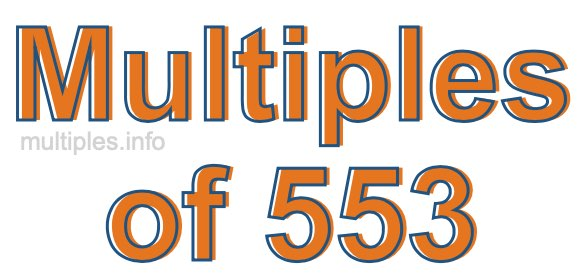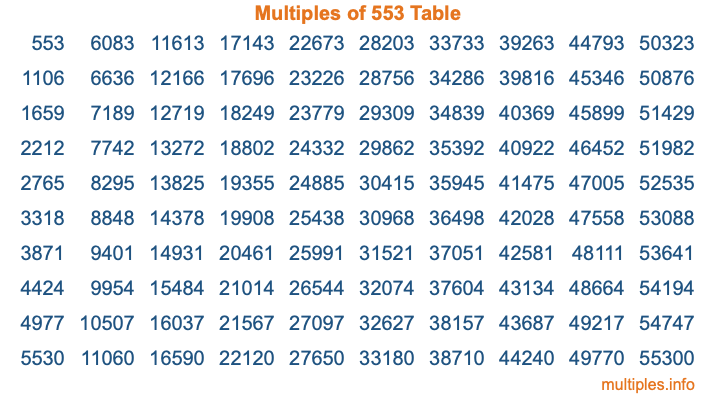Multiples of 553Welcome to the Multiples of 553 page. Here we will first teach you everything you will ever need to know about the multiples of 553, and then give you a study guide summary of everything we taught you to make sure you remember it all. Use this page to look up facts and learn information about the multiples of 553. This page will make you a multiples of five hundred fifty-three expert!

Definition of Multiples of 553
Multiples of 553 are all the numbers that when divided by 553 equal an integer. Each of the multiples of 553 are called a multiple. A multiple of 553 is created by multiplying 553 by an integer.

Therefore, to create a list of multiples of 553, you start with 1 multiplied by 553, then 2 multiplied by 553, then 3 multiplied by 553, and so on for as long as you want. Thus, the list of the first five multiples of 553 is 553, 1106, 1659, 2212, and 2765. To see a larger list of multiples of 553, see the printable image of Multiples of 553 further down on this page. We also have a category where you can choose any nth multiple of 553.

Multiples of 553 Checker
The Multiples of 553 Checker below checks to see if any number of your choice is a multiple of 553. In other words, it checks to see if there is any number (integer) that when multiplied by 553 will equal your number. To do that, we divide your number by 553. If the the quotient is an integer, then your number is a multiple of 553.

Is  a multiple of 553?

Least Common Multiple of 553 and ...
A Least Common Multiple (LCM) is the lowest multiple that two or more numbers have in common. This is also called the smallest common multiple or lowest common multiple and is useful to know when you are adding our subtracting fractions. Enter one or more numbers below (553 is already entered) to find the LCM.

Check out our LCM Calculator if you need more details about the Least Common Multiple or if you need the LCM for different numbers for adding and subtraction fractions.

nth Multiple of 553
As we stated above, 553 is the first multiple of 553, 1106 is the second multiple of 553, 1659 is the third multiple of 553, and so on. Enter a number below to find the nth multiple of 553.

th multiple of 553

Multiples of 553 vs Factors of 553
553 is a multiple of 553 and a factor of 553, but that is where the similarities end. All postive multiples of 553 are 553 or greater than 553. All positive factors of 553 are 553 or less than 553.

Below is the beginning list of multiples of 553 and the factors of 553 so you can compare:

Multiples of 553: 553, 1106, 1659, 2212, 2765, etc.

Factors of 553: 1, 7, 79, 553

As you can see, the multiples of 553 are all the numbers that you can divide by 553 to get a whole number. The factors of 553, on the other hand, are all the whole numbers that you can multiply by another whole number to get 553.

It's also interesting to note that if a number (x) is a factor of 553, then 553 will also be a multiple of that number (x).

Multiples of 553 vs Divisors of 553
The divisors of 553 are all the integers that 553 can be divided by evenly. Below is a list of the divisors of 553.

Divisors of 553: 1, 7, 79, 553

The interesting thing to note here is that if you take any multiple of 553 and divide it by a divisor of 553, you will see that the quotient is an integer.

Multiples of 553 Table
Below is an image of the first 100 multiples of 553 in a table. The table is in chronological order, column by column. The first column has the first ten multiples of 553, the second column has the next ten multiples of 553, and so on.The Multiples of 553 Table is also referred to as the 553 Times Table or Times Table of 553. You are welcome to print out our table for your studies.

Negative Multiples of 553
Although not often discussed or needed in math, it is worth mentioning that you can make a list of negative multiples of 553 by multiplying 553 by -1, then by -2, then by -3, and so on, to get the following list of negative multiples of 553:

-553, -1106, -1659, -2212, -2765, etc.

Multiples of 553 Summary
Below is a summary of important Multiples of 553 facts that we have discussed on this page. To retain the knowledge on this page, we recommend that you read through the summary and explain to yourself or a study partner why they hold true.

There are an infinite number of multiples of 553.

A multiple of 553 divided by 553 will equal a whole number.

553 divided by a factor of 553 equals a divisor of 553.

The nth multiple of 553 is n times 553.

The largest factor of 553 is equal to the first positive multiple of 553.

553 is a multiple of every factor of 553.

553 is a multiple of 553.

A multiple of 553 divided by a divisor of 553 equals an integer.

553 divided by a divisor of 553 equals a factor of 553.

Any integer times 553 will equal a multiple of 553.

Multiples of a Number
Here you can get the multiples of another number, all with the same attention to detail as we did for multiples of 553 on this page.

Multiples of
Multiples of 554
Did you find our page about multiples of five hundred fifty-three educational? Do you want more knowledge? Check out the multiples of the next number on our list!